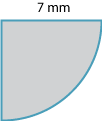#### Question 5

 The circumference of a circle with radius 14 cm is: 7π cm 14π cm 28π cm

#### Question 6

 The circumference of a circle with diameter 14 cm is: 7π cm 14π cm 28π cm

#### Question 7The approximate value of the perimeter of the figure shown, using $$\pi \approx \dfrac{22}{7}$$ is: 11 mm 18 mm 25 mm